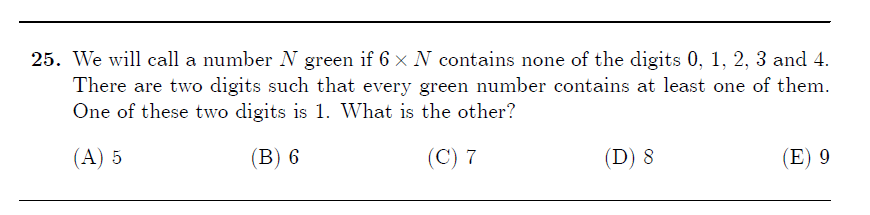# Help: Digits question

• Hi ,Can anyone help to work out how to solve this question? Thanks• Hey @pioneeringemu!

Here's a solution that uses the choices: can you think of any multiples of $$6$$ that doesn't use any of the digits 0-4? Try and list out a few. Once you have a multiple 6 that satisfies that, that's a possibility for $$6N$$. Can you figure out which one is the answer now?

While you try that out, I'll work out a solution that is more precise• @quacker88 thanks

• @pioneeringemu I ended up finishing a more legit solution, here it is just in case other people stop by this post and want to see it too:

A number $$N$$ is green if $$6N$$ has none of the digits $$0,1,2,3,4$$.

Looking at one-digit numbers, right away we know that $$1$$ is a green number. That doesn't help though, since the problem already tells us that every green number has either a $$1$$ or some other digit in it. Now, let's consider two-digit numbers.

Let's start off by considering the units digits of $$6N$$.

If $$N$$ has units digit 1, then $$6N$$ has units digit 6.
If $$N$$ has units digit 2, then $$6N$$ has units digit 2.
Filling it out for all the digits, we have
1 -> 6
2 -> 2
3 -> 8
4 -> 4
5 -> 0
6 -> 6
7 -> 2
8 -> 8
9 -> 4

Using this list, we know that all green numbers must end in 1, 3, 6, or 8.

A quick check though shows that out of 1, 3, 6, and 8, only 1 is a green number. So this confirms that green numbers do indeed have to contain a 1 or some other number, but the problem already gave us that.
So, let's start looking through the two-digit numbers.

The possible two-digit green numbers are going to look like $$A1, A3, A6, A8$$.
*note: whenever I use $$A$$ here, I mean it as a digit. $$A1$$ is a number with $$A$$ in the tens place and $$1$$ in the ones place, not $$A\cdot1$$.

Now, let's see what $$6$$ times these numbers gives us.This is what $$A1 \cdot 6$$ looks like. There is no digit $$A$$ for which this works, because no value of $$6A$$ has none of the digits $$0,1,2,3,4$$. Let's move on to the case of $$A3 \cdot 6$$.So, we need a digit $$A$$ such that $$6A+1$$ has none of the digits $$0\to4$$. Wait, $$A=9$$ works! $$93\cdot6=558$$, which has no digits $$0\to4$$, so $$93$$ is a green number (note that $$A=1$$ also works in this case too, but that doesn't help us because the problem already tells us that $$1$$ is one of the two numbers).

We already have two green numbers now: $$1$$ and $$93$$. Since the problem says that every green number has either a $$1$$ or some other digit in it, we now know that the other digit has to be $$9$$ or $$3$$. Since the answer choices has no option for $$3$$, the answer has to be $$\boxed{(E)\text{ } 9}$$.

But, what if they didn't give us the answer choices? Well, I guess we just have to keep going through the cases.Here's what $$A6 \cdot 6$$ looks like. Again, the only possible $$A$$ are $$A=1,9$$. And we can confirm, $$16$$ and $$96$$ are green numbers, since $$16\cdot6=96$$ and $$96\cdot6=576$$. That means that the two numbers are indeed $$1$$ and $$9$$, and we can be safe with E as our answer.